# Applying PCA on MNIST dataset

## Introduction

Before we learn about the MNIST dataset and dive deeper into the code, we must recap Principal Component Analysis (PCA).

Principal Component Analysis (PCA) is a dimensionality reduction technique that helps us convert a high dimensional dataset(a data set with lots of features/variables) into a low dimensional dataset. PCA helps us prevent our machine learning model from overfitting on our data and enables us to retain information from all the significant variables for our model.

PCA is also used to reduce dimensionality so that we can easily visualize the data.
PCA uses feature extraction, i.e., it combines our input variables in a specific way so we can drop the least important or least significant variables while still retaining the fundamental attributes of our old variables.

PCA takes the variance(or spread) of the data into account to reduce the dimensions.  Dimensions or variables having high variance have high information. Therefore, variables having very low variance can be removed or skipped.

When the variance of both the dimensions is comparable, i.e., there is no significant difference in the variance of the dimensions we are trying to compare, PCA projects the data from the two dimensions into a single vector which is the direction of the maximum variance. Let's understand this with an example.

Let us assume that we have a two-dimensional dataset, and we want to reduce the data into just one dimension. The scatter plot obtained from plotting the data is the image below.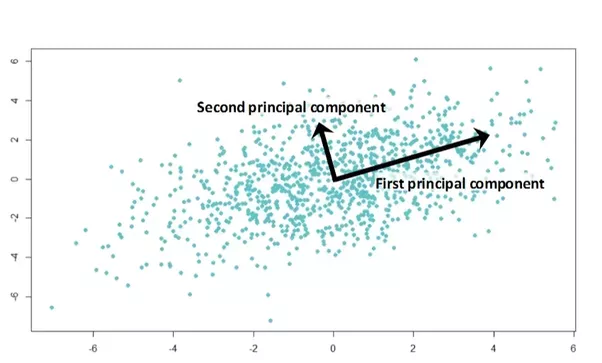Source: Medium

Now two principal components are calculated. The first principal component is the one in which the data variance is maximum. The second is the component with the second-highest variance in data and perpendicular to the first principal component. The number of components calculated equals the number of dimensions to which our data would be reduced. Since we want to reduce it to just one dimension, we take a projection of our data points along the first principal component( maximum variance) and thus obtain a new single feature.

Therefore PCA can be broken down into five steps:

1. Standardization of the range of continuous initial variables
2. Computation of the covariance matrix to identify correlations
3. Calculation of the eigenvectors and eigenvalues of the covariance matrix to identify the principal components
4. Creation of a feature vector to decide which principal components to keep
5. Recasting the data along the axes of the principal components.

Now we will see how we can implement PCA in code as we will be applying PCA on the MNIST dataset.

## MNIST Dataset

The MNIST(Modified National Institute of Standards and Technology database) is a subset of an extensive database of handwritten digit images used for training various Machine Learning models.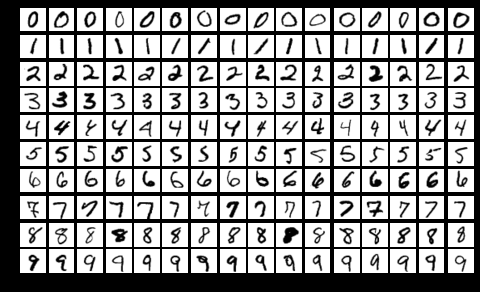Source: Wikipedia

No. of training images: 60,000

No. of testing images: 10,000

Half of the training set and half of the test were taken from NIST's training dataset, while the other half of both respectively were taken from NSIT's testing dataset.

Each image is 28x28 pixels. Each pixel value is between 0 to 255, which denotes the lightness or darkness of that pixel.

The training data set(.csv) has 785 columns. The first column denotes the label, i.e., which digit has been drawn, and the rest of the 784 values denote the value of the pixels in the image.

## Implementation

In this section, we will reduce the 784 dimensions of the MNIST dataset to 2 dimensions and plot the corresponding principal components obtained.

Let's start by importing the basic libraries.

``````import numpy as np
import pandas as pd
import seaborn as sns``````

Now let’s load the data into a pandas dataframe. Give the path to the train.csv file in your system for the read_csv function.

``````data=pd.read_csv("D:/mnist/train.csv") #load the data into a pandas dataframe
data.head(5) #Show the first 5 rows``````

The output is given below(first five rows)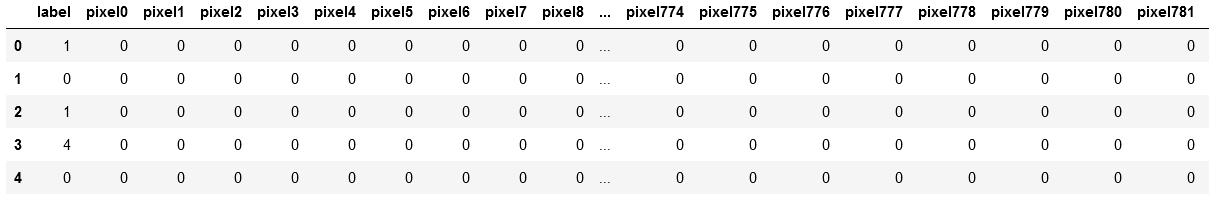Now let’s drop the label column so that we only have the features i.e the pixel values in our dataset.

``````label=data['label'] # save label data for later use
data.drop('label', axis = 1, inplace = True)``````

Before applying PCA, it is essential to standardize our dataset, i.e., the mean should be 0, and the standard deviation should be 1 for each variable. We can scale our data with the help of the StandardScaler method in sklearn.

``````from sklearn.preprocessing import StandardScaler
data_standardized = StandardScaler().fit_transform(data)``````

Now we need to compute the covariance matrix, which helps us determine the relationship between the dimensions. The covariance matrix for a matrix can be calculated by multiplying the matrix with its transpose.

``covMatrix = np.matmul(data_standardized.T ,data_standardized) # matrix multiplication in numpy``

Now we will compute the eigenvalues and the eigenvector, which would help us determine the principal components. We will use the linear algebra library in scipy(linalg) to compute the eigenvalues.

``````from scipy.linalg import eigh
values, vector = eigh(covMatrix,eigvals=(782,783))
values``````

The eigh function returns eigenvalues in ascending order. Therefore if we need the highest two, we have to give the tuple (783,784), which means that values will contain the 2nd principal component and values will contain the 1st principal component.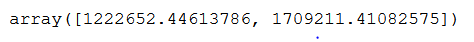We will transpose the eigenvector to make it easy to use it further.

``````print("The shape before",vector.shape)
vector=vector.T
print("The new shape",vector.shape)``````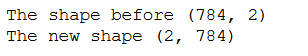Now we will project the original data on the vector plane formed using vector multiplication.

``projectedData = np.matmul(vector, data_standardized.T)``

Let’s stack our projected data with labels so that we have our final dataframe ready for visualization.

``````reducedData = np.vstack((projectedData, label)).T #Stack with labels
reducedData = pd.DataFrame(reducedData, columns = ['pca_1', 'pca_2', 'label'])``````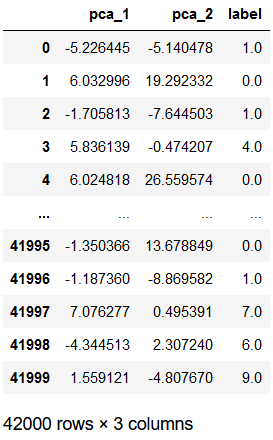Now lets plot the data with the help of seaborn Facetgrid method.

``````sns.FacetGrid(reducedData, hue = 'label', size = 8) \
.map(sns.scatterplot, 'pca_1', 'pca_2') \

Therefore we have converted our dataset from 784 dimensions to 2 dimensions.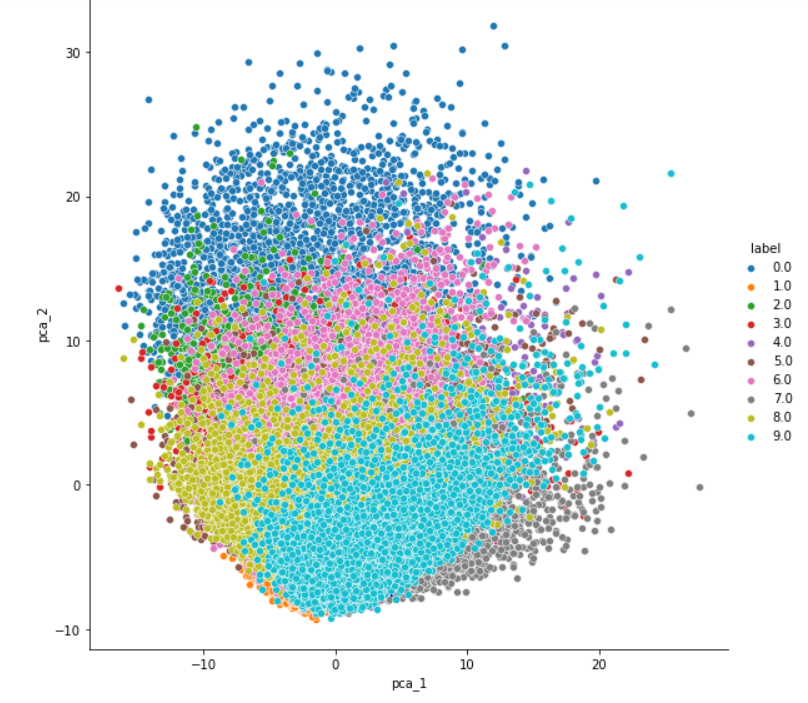1. What is the need for standardization of data in PCA?
PCA gives more emphasis to variables with high variance. Therefore, if the dimensions are not scaled, we will get inconsistent results. For example, the value for one variable might lie in the range 50-100 and the other one 5-10. In this case, PCA will give more weight to the first variable. Such issues can be resolved by standardizing the dataset before applying PCA

2. What is FacetGrid in seaborn?
FacetGrid is used for plotting conditional relationships. The basic workflow is to initialize the FacetGrid object with the dataset, and the variables used to structure the grid. Then one or more plotting functions can be applied to each subset by calling FacetGrid.map() or FacetGrid.map_dataframe(), and then the other customizations can also be done. I recommend you to look at the documentation for more details.

3. How can I plot an image from the MNIST dataset?
After loading the data, we can easily plot the image with the help of the pixel values. The code snippet is given below.

``````import matplotlib.pyplot as plt
index=1234 #Select a random index
fig_data = np.array(data.iloc[index]).reshape(28,28) #Reshape it into the size 28x28
plt.imshow(fig_data, interpolation = None, cmap = 'gray') #Use imshow function from pyplot to plot the figure
plt.show()
print(label[index])``````

## Key Takeaways

We learned that we could apply PCA on high-dimensional datasets and reduce them to low dimensions. We have also learned how to use python effectively to load our datasets, apply PCA on them, and prepare our data for future machine learning models.